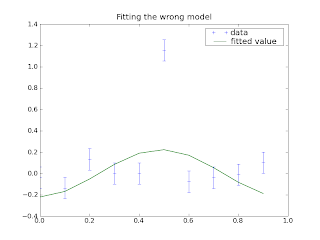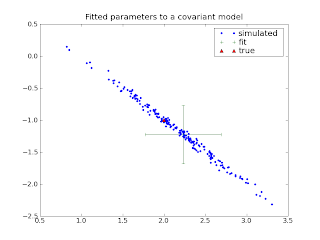### Curve fitting, part 2: Quality control the dumb wayWhen you're fitting a curve to data, it's important to know not just which curve fits the data most closely, but also how well the data constrains the curve, and how good a fit the curve actually is. This is plainly impossible if you don't know how good your data is, and estimating the uncertainties on experimental data is often very difficult, but I'm going to assume for the moment that you have done that. The problem now is to understand the statistics of the fitting process. For the moment, I'm going to continue using the simple linear least-squares example I introduced in part 1.

In part 1, we found the parameters that minimized the sum of squares of differences between the data and our model. Now let's ask how close we can expect those parameters to be to the true parameters. There are statistics to do this quickly and conveniently, but for the moment I'm going to use the "everything looks like a nail" approach of direct simulation: I'll generate, say, 1000 fake data sets, and I'll fit a model to each one, and I'll record the parameters. Then I'll look at the distribution of these fitted parameters. (A brief aside: if you don't care about the details, just read right past these blocks of code. The text should be reasonably clear without them.)
def fit_many_parameters(model, parameters, fit_function, n):    result = np.zeros((n,len(parameters)))    for i in range(n):        fake_data = model.generate(parameters)        result[i] = fit_function(model, fake_data)    return result

Since in general we don't have access to the true model that generated our real data, I'm going to do this starting from our best-fit model. This is making the assumption that the errors in our fitting procedure are the same around the best-fit model are the same as the errors around the true model. Since we are doing linear fitting, this is exactly true, but in general it is only approximately true. But the degree to which it can be wrong is basically the degree to which your best-fit function differs from the true one, so it shouldn't be a problem unless you have a horrible fit. In this case you'll have to be more clever.
def plot_many_parameters(model, true_parameters):    data = model.generate(true_parameters)    fit_parameters = fit_linear_least_squares(model, data)    varied_parameters = fit_many_parameters(model, fit_parameters,             fit_linear_least_squares, 200)    plt.figure()    plt.plot(varied_parameters[:,0], varied_parameters[:,1],             ".", label="simulated")    plt.errorbar([fit_parameters],[fit_parameters],            xerr=np.std(varied_parameters[:,0]),            yerr=np.std(varied_parameters[:,1]),            fmt="+", label="fit")    plt.plot([true_parameters],[true_parameters],"^", label="true")    plt.legend(loc="best")def demo_least_squares_uncertainties():    true_parameters = np.array([2.,-1.])    n = 10    uncertainty = 0.1    model = SinCosModel(n,uncertainty)    plot_many_parameters(model, true_parameters, n, uncertainty)    plt.title("Fitted parameters to a sin/cos model")    plt.savefig("monte-carlo-errors-1.png")

The result:You get a cloud of values, with the true value not in the center, but not too far out. It's tempting (and not totally unreasonable) to simply write down the standard deviations of each parameter as the uncertainties. But we were lucky. Let's try writing the model a little differently and running it again:
class CovariantSinusoidModel(SinCosModel):    def predicted_values(self, parameters):        a, b = parameters        xs = np.arange(self.n)/float(self.n)        return a*np.sin(2*np.pi*xs) + b*np.sin(2*np.pi*xs+0.1)def demo_least_squares_uncertainties_covariant():    true_parameters = np.array([2.,-1.])    n = 10    uncertainty = 0.1    model = CovariantSinusoidModel(n,uncertainty)    plot_many_parameters(model, true_parameters)    plt.title("Fitted parameters to a covariant model")    plt.savefig("monte-carlo-errors-2.png")Now the "cloud" of values is long and skinny and not aligned with either axis. Either parameter can vary by a lot, but if one is too high the other is almost certain to be too low - simply using standard deviations just doesn't describe this distribution well at all. The right answer is to write down a "correlation matrix" that describes not just the spread in each parameter but how much they're correlated with each other.

Describing these errors using standard deviations and a correlation matrix like this has the advantage that if you ignore the correlation matrix, you get a pessimistic estimate of the errors: You throw away the knowledge that the real answer lies on that nice little diagonal and you get a much larger error circle, but at least the real answer will still be in your error circle. Errors too big: weaker claims. Errors too small: serious embarrassment when someone finds the real answer isn't what you claimed.

It's worth pointing out that in this case, our parameters describe exactly the same set of solutions as the first did: any sinusoid can be expressed as a linear combination of our two sinusoids. The problem is just that our parameters don't describe the space very well. So when possible, it's better still to reparameterize in a way that removes as much of the correlation as possible. This particular example is contrived, but if you were fitting, say, a straight line to your data, you'd have two parameters, slope and vertical offset. If you choose the vertical offset measured at some arbitrary zero, you will probably have some huge covariance like we see here. You can reduce it drastically by measuring the offset at the middle of your data set. If you can, this is a better solution than just living with the covariance.

Now what about quality-of-fit? Our basic approach is going to be simulation again (to be improved soon, I promise). We'll devise some measure of goodness of fit, then we'll generate another batch of fake data sets, and we'll ask how many of them are a better fit than ours. After all, there's noise in there, so we wouldn't expect a perfect fit. But if our data is more different from the model than a thousand randomly-generated data sets, either we were really unlucky or our model is wrong.

The goodness-of-fit number we're going to use is called the chi-squared, and it's the sum of (ith data value - ith predicted value)**2/(ith uncertainty)**2.
def chi2(data, predicted, uncertainties):    return np.sqrt(np.sum(((data-predicted)/uncertainties)**2,axis=-1))

So first let's do this for data coming from the right kind of model:
def plot_quality_of_fit(model, parameters, data, n_samples=1000):    data_chi2 = chi2(data,                model.predicted_values(parameters),                 model.uncertainties(parameters))       chi2_values = np.zeros(n_samples)    for i in range(n_samples):        chi2_values[i] = chi2(model.generate(parameters),                 model.predicted_values(parameters),                 model.uncertainties(parameters))    chi2_values.sort()    n_less = np.searchsorted(chi2_values, data_chi2)    plt.figure()    # make a "stair-step" plot    chi2_r = np.repeat(chi2_values,2)    y2 = np.hstack([0.0,         np.repeat(np.arange(1, n_samples)/float(n_samples),2),        1.0])    plt.plot(chi2_r, y2)    plt.axvline(data_chi2, color='red')    plt.axhline(n_less/float(n_samples), color='red')    plt.text(1,0,            ("a fraction %g of the samples are this bad" %                 (1-n_less/float(n_samples))),            horizontalalignment="right",            verticalalignment="bottom",            transform = plt.gca().transAxes)    plt.xlabel("$\chi^2$")    plt.ylabel("Fraction of samples less than given $\chi^2$")    def demo_goodness_of_good_fit():    true_parameters = np.array([2.,-1.])    n = 10    uncertainty = 0.1    model = SinCosModel(n,uncertainty)    data = model.generate(true_parameters)    fit_parameters = fit_linear_least_squares(model, data)    plot_quality_of_fit(model, fit_parameters, data)    plt.title("Goodness of fit for a correct model")    plt.savefig("monte-carlo-good-fit.png")Now let's make a really different model, and use it to generate our original data set, but fit the same sinusoidal model to it:
def demo_goodness_of_bad_fit():    n = 10    uncertainty = 0.1    xs = np.arange(n)/float(n)    model = SinCosModel(n,uncertainty)    data = np.random.normal(scale=uncertainty,size=n)    data[n//2] += 1.    fit_parameters = fit_linear_least_squares(model, data)    plt.figure()    plt.errorbar(xs, data, uncertainty, label="data", fmt="+")    plt.plot(xs,model.predicted_values(fit_parameters), label="fitted value")    plt.xlim(0,1)    plt.title("Fitting the wrong model")    plt.legend(loc="best")    plt.savefig("wrong-model.png")    plot_quality_of_fit(model, fit_parameters, data, n_samples=10000)    plt.title("Goodness of fit for an incorrect model")    plt.savefig("monte-carlo-bad-fit.png")

This produces the fit seen at the top of the article:And sure enough it's a bad fit:This business of simulation is very simple, but it has important limitations. One is visible in this last plot: our model is such a bad fit not one random example was as bad as the real data set. So we have no way to judge the significance more closely than "less than about one in ten thousand". Often we'd like to know whether it's a one-in-a-million - after all, maybe we searched through a million possibilities to find it - or one in 10^40. Without running really outrageous numbers of simulations, we can't find this information without doing some analytical work. Fortunately in this case the statistics are pretty simple, and I'll talk about them next time.